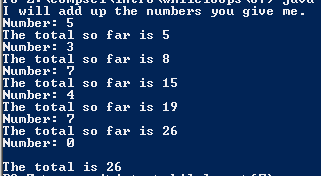# Assignemnt #67 and Adding Values in a Loop

## Code

```/// Name: Raff Lisbona
/// Period: 6
/// Date Finished: 12/2/2015

import java.util.Scanner;
{
public static void main( String[] args )
{
int number, sum = 0;

Scanner keyboard = new Scanner(System.in);

System.out.println("I will add up the numbers you give me.");
System.out.print("Number: ");
number = keyboard.nextInt();

while ( number != 0 )
{
sum = sum + number;
System.out.println("The total so far is " + sum);
System.out.print("Number: ");
number = keyboard.nextInt();
}
System.out.println(" ");
System.out.println("The total is " + sum);
}
}
```

### Picture of the output